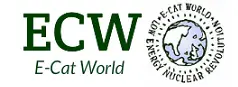# E-capture (Gerhard Hunf)

The following post has been submitted by Gerhard Hunf

“E-capture”

In “ https://www.e-catworld.com/2016/11/15/high-current-densities-environmental-conditions-for-cold-fusion-gerhard-hunf/ “ was shown, the current flow at the contact point (conductor / superconductor) is almost completely changed from the conductor to the superconductor. For this, the velocity of the electrons must change in the ratio of the cross sections mm2 / nm2 (conductor / superconductor). The branching point (conductor / superconductor) thus acts as a particle accelerator for electrons. The electron becomes heavier.

The “e-capture reaction” can be formulated as follows
(p + + e) + E1 = n + v.

E1 can be calculated from the mass difference Δm = m (neutron) – m (p + + e). The mass difference is 1.39 x 10exp-30 kg. Substituting this into E mc2 gives E1 = 1.249x10exp-13J or 0.78MeV.

This work W = U I t. must be supplied by the system. In order to check whether a current density (J) of 10exp11 A / m2 is sufficient, we use for the superconducting layer
W = U I t = U x (J x F) x s / v => J = W x v / U x F x s

W = 1.249×10-exp-13J; v = 10exp2 m / sec; U = 12V (battery); F = 10exp-12 m2; s = 10exp-10 m. ! Attention: 1Volt is also 1J/C, 1Ampere is also 1C/sec!

From this follows current density (J) is about 10exp11 A / m2

This means current density for the “e-capture” is the same achieved in the superconducting layer.

Gerhard Hunf